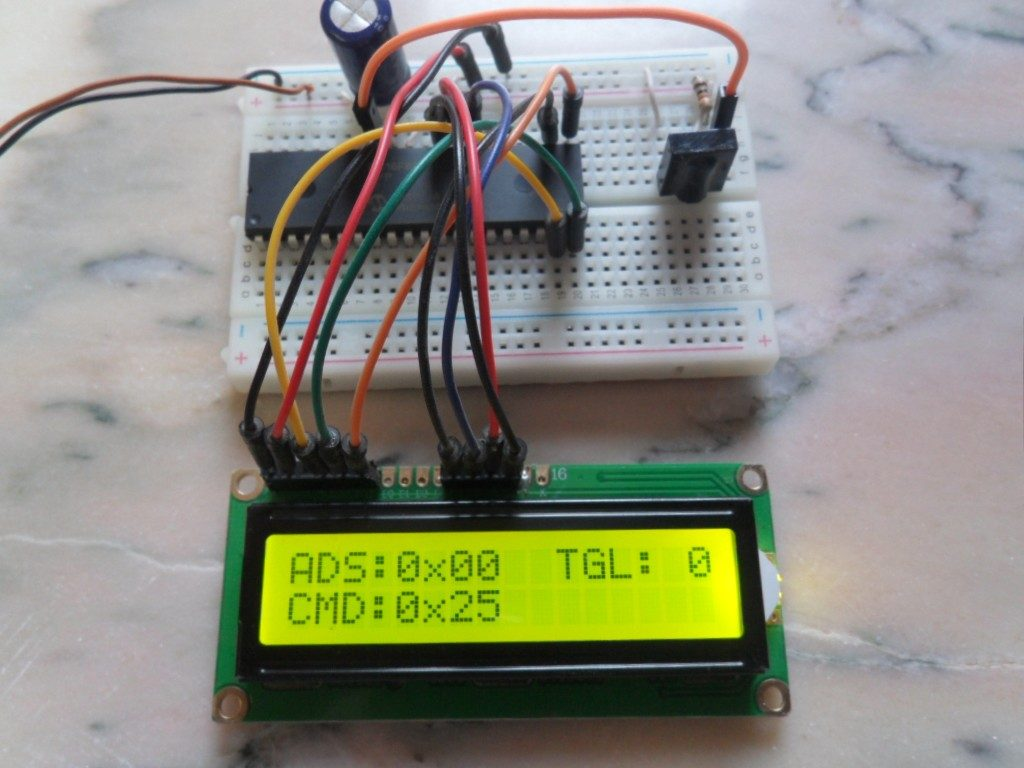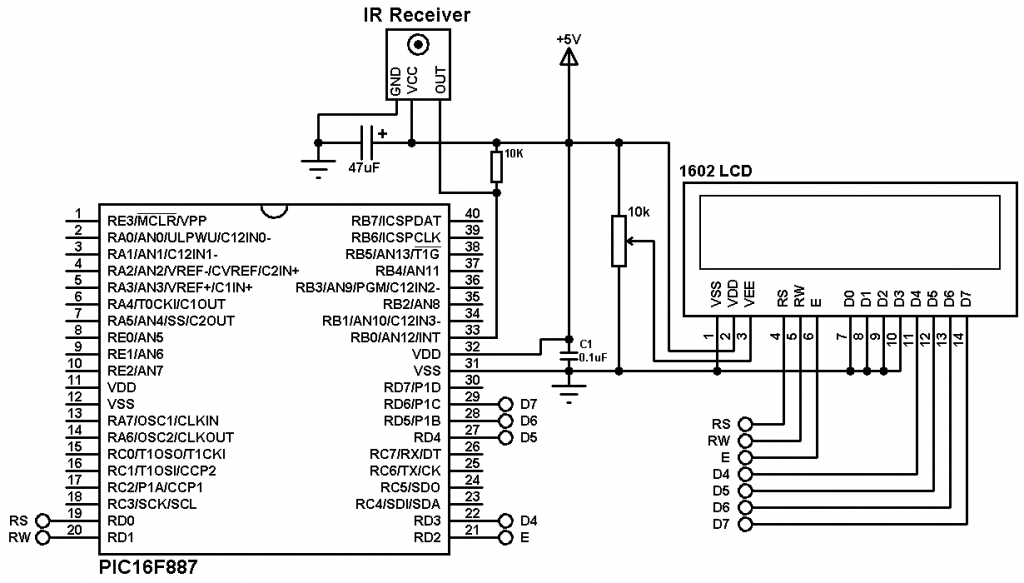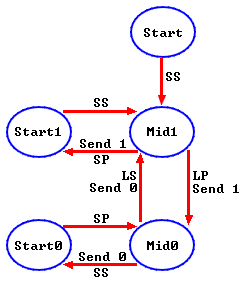# RC-5 Decoder with PIC16F887 microcontroller and CCS C compiler

This article shows how to decode IR remote controls which use Philips RC-5 protocol using PIC16F887 microcontroller and CCS PIC C compiler where the results are displayed on 1602 LCD screen.

The RC5 has 14 bits per 1 code transmission, the 14 bits can be divided into 4 parts:
The first 2 bits are start bits and they are always logic 1.
The third bit called toggle bit, it can be logic 1 or logic 0.
The next 5 bits are address bits, each device type has its address number for example TV address number is 0, CD player address = 20 …………
And the last 6 bits are command bits, each button has its command number.
For the same device for example TV all the remote control buttons has the same address but each button has its command.
The toggle bit changes whenever a button is pressed.

The RC5 protocol uses Manchester coding, a logic 0 is represented by a logic high in the first half and a logic low in the second half, whereas a logic 0 is represented by a logic low in the first half and a logic high in the second half. The length of each half is 889µs which means the length of 1 bit is 1778µs.

Hardware required:

• PIC16F887 microcontroller
• RC5 IR remote control
• 16×2 LCD screen
• 47µF capacitor
• 0.1µF ceramic capacitor (optional)
• 10K ohm variable resistor
• 10K ohm resistor
• 5V Power source
• Protoboard
• Jumper wiresRC5 Decoder with PIC16F887 circuit:PIC16F887 uses its internal oscillator which is configured in the C code.
As shown in the circuit diagram, the output of the IR receiver is connected to external interrupt pin which is RB0. A 10K ohm resistor is connected between the IR receiver output and +5V in order to minimize noise which comes from it.

RC-5 Decoder with PIC16F887 CCS C code:
Before writing the C code of the decoder, I drew a simple state machine of the RC5 protocol which helped me a lot in the code. The state machine is shown below.
I made the code as simple and small as I can.Where:
SP : Short Pulse (About 889µs)
LP : Long Pulse (About 1778µs)